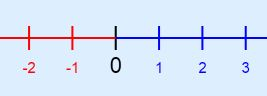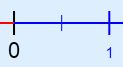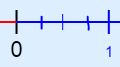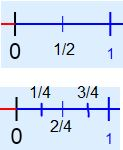# How to Find Equivalent Fractions on a Number Line

Instructor: Yuanxin (Amy) Yang Alcocer

Amy has a master's degree in secondary education and has taught math at a public charter high school.

Your number line can actually help you figure out equivalent fractions if you know what steps to take to break up your number line in the right way. Learn how in this how-to lesson.

## The Steps

In this lesson, we'll talk about equivalent fractions and how using a number line can help us find them. Our equivalent fractions are different ways to write the same fraction. For example, 1/2 and 2/4 are equivalent fractions. If you simplify 2/4 by dividing both the numerator and denominator by 2, you'll get 1/2, the same as the other one.

• 1/2 = 2/4

Remember, our number line is a line with evenly spaced tick marks that show us our numbers.To work with equivalent fractions, we begin with one fraction such as 1/2. To find the equivalent fractions, we'll follow these steps.

#### Step 1: Locate the original fraction on the number line.

We'll first need to locate our fraction on the number line. To do this, you'll need to divide the first tick mark into even pieces. If you are looking for the fraction 1/2, you'll need to divide the first tick mark (the space between the number 0 and 1) into two even pieces. If you are looking for 1/3, then you'll need to divide the first tick mark into three even pieces. So, the denominator tells you how many even pieces to divide that first tick mark into and the numerator tells you which of those tick marks your fraction is. Place a small tick mark to mark your dividers.

So, 1/2 has you dividing your first tick mark into two even spaces. You place a small tick mark in the middle of your line between the number 0 and the number 1. The numerator 1 tells you that your fraction is the first of the small tick marks (the only small tick mark in this case).#### Step 2: Divide each your newly created divisions from step 1 into two even spaces.

Now, to find our equivalent fractions, we'll need to divide the divisions we made from step 1 into two even spaces.

So, for your 2/4, you'll be dividing the two divisions you made in step 1 in half. So you'll have four equal spaces between your number 0 and your number 1 now.#### Step 3: Find the equivalent fraction.

To find out what our equivalent fraction is, we'll now need to read our number line.

For your 1/2, you remember that the 1/2 is the middle separation between the number 0 and the number 1. But for this number line, you now have four separations. So this means the denominator is now 4. The middle line between 0 and 1 is the second small tick mark, so the numerator is 2. This means that 1/2 and 2/4 are equivalent fractions.#### Step 4: Repeat steps 2 and 3 to find more equivalent fractions.

If you want to find more equivalent fractions, keep repeating steps 2 and 3.

## The Solution

You've found your answer to be 2/4. An equivalent fraction to 1/2 is 2/4.

To unlock this lesson you must be a Study.com Member.

### Register to view this lesson

Are you a student or a teacher?

#### See for yourself why 30 million people use Study.com

##### Become a Study.com member and start learning now.
Back
What teachers are saying about Study.com

### Earning College Credit

Did you know… We have over 200 college courses that prepare you to earn credit by exam that is accepted by over 1,500 colleges and universities. You can test out of the first two years of college and save thousands off your degree. Anyone can earn credit-by-exam regardless of age or education level.